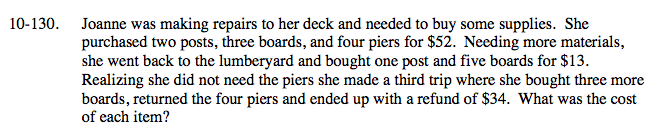### Home > A2C > Chapter Ch10 > Lesson 10.2.5 > Problem10-130

10-130.b = cost of boards ($) p = cost of posts ($) i = cost of piers ($) 2p + 3b + 4i = 52 p + 5b = 13 3b − 4i = −34 Now, solve the system of equations using elimination. The boards cost$2, the posts cost $3, and the piers cost$10.